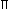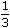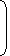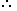# Tricks to solve Volume and Surface Area Problems1. CUBOID
Let length = l, breadth = b and height = h units. Then

1. Volume = (l x b x h) cubic units.
2. Surface area = 2(lb + bh + lh) sq. units.
3. Diagonal = l2 + b2 + h2 units.
2. CUBE
Let each edge of a cube be of length a. Then,

1. Volume = a3 cubic units.
2. Surface area = 6a2 sq. units.
3. Diagonal = 3a units.
3. CYLINDER
Let radius of base = r and Height (or length) = h. Then,

1. Volume = (r2h) cubic units.
2. Curved surface area = (2rh) sq. units.
3. Total surface area = 2r(h + r) sq. units.
4. CONE
Let radius of base = r and Height = h. Then,

1. Slant height, l = h2 + r2 units.
2. Volume =r2hcubic units.
3. Curved surface area = (rl) sq. units.
4. Total surface area = (rl +r2) sq. units.
5. SPHERE
Let the radius of the sphere be r. Then,

1. Volume =r3cubic units.
2. Surface area = (4r2) sq. units.
6. HEMISPHERE
Let the radius of a hemisphere be r. Then,

1. Volume =r3cubic units.
2. Curved surface area = (2r2) sq. units.
3. Total surface area = (3r2) sq. units.Note: 1 litre = 1000 cm3.
A right triangle with sides 3 cm, 4 cm and 5 cm is rotated the side of 3 cm to form a cone. The volume of the cone so formed is:
 A. 12cm3 B. 15cm3 C. 16cm3 D. 20cm3

Explanation:Clearly, we have r = 3 cm and h = 4 cm.Volume = 1r2h =1 xx 32 x 4cm3 = 12cm3. 3 3
In a shower, 5 cm of rain falls. The volume of water that falls on 1.5 hectares of ground is:
 A. 75 cu. m B. 750 cu. m C. 7500 cu. m D. 75000 cu. m

Explanation:

1 hectare = 10,000 m2

So, Area = (1.5 x 10000) m2 = 15000 m2.

 Depth = 5 m = 1 m. 100 20Volume = (Area x Depth) =15000 x 1m3 = 750 m3. 20

#### A hall is 15 m long and 12 m broad. If the sum of the areas of the floor and the ceiling is equal to the sum of the areas of four walls, the volume of the hall is:

Correct! Wrong!

#### 66 cubic centimetres of silver is drawn into a wire 1 mm in diameter. The length of the wire in metres will be:

Correct! Wrong!

#### A hollow iron pipe is 21 cm long and its external diameter is 8 cm. If the thickness of the pipe is 1 cm and iron weighs 8 g/cm3, then the weight of the pipe is:

Correct! Wrong!

#### A boat having a length 3 m and breadth 2 m is floating on a lake. The boat sinks by 1 cm when a man gets on it. The mass of the man is:

Correct! Wrong!

#### 50 men took a dip in a water tank 40 m long and 20 m broad on a religious day. If the average displacement of water by a man is 4 m3, then the rise in the water level in the tank will be:

Correct! Wrong!

QUIZ ON VOLUME & SURFACE AREAS
GREAT JOB!!!!!